Function Repository Resource:

# RationalCholeskyDecomposition

Compute the rational Cholesky decomposition of a matrix

Contributed by: Jan Mangaldan
 ResourceFunction["RationalCholeskyDecomposition"][m] gives the rational Cholesky decomposition of a matrix m, given as a list {l,d} where l is a unit lower-triangular matrix and d is the diagonal of a diagonal matrix.

## Details

The rational Cholesky decomposition is also referred to as the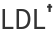decomposition.
The matrix m can be numerical or symbolic, but must be Hermitian and positive definite.
ResourceFunction["RationalCholeskyDecomposition"][m] yields a lower‐triangular matrix l and a list d so that l.DiagonalMatrix[d].ConjugateTranspose[l]m.

## Examples

### Basic Examples (2)

Perform a rational Cholesky decomposition on a matrix m:

 In:=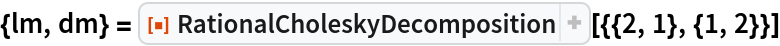Out=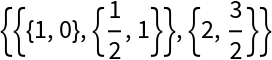Confirm that lm.DiagonalMatrix[dm].ConjugateTranspose[lm]m:

 In:=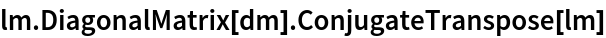Out=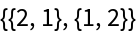### Scope (3)

Hilbert matrices are symmetric and positive definite:

 In:=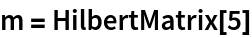Out=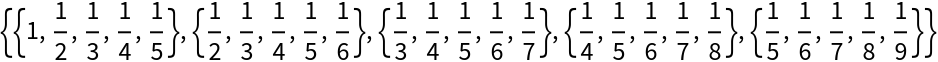Compute the rational Cholesky decomposition with exact arithmetic:

 In:=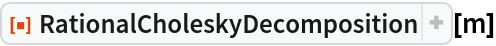Out=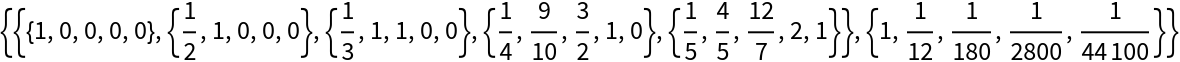In:=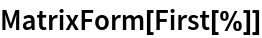Out=Compute the rational Cholesky decomposition with machine arithmetic:

 In:=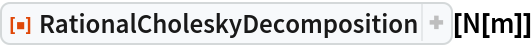Out=Compute the rational Cholesky decomposition with 24-digit precision arithmetic:

 In:=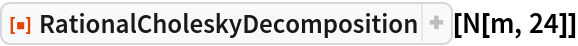Out=Compute the rational Cholesky decomposition of a random complex Hermitian matrix:

 In:=Out=Use symbolic matrices:

 In:=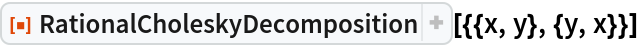Out=### Properties and Relations (4)

Create a symmetric positive definite matrix:

 In:=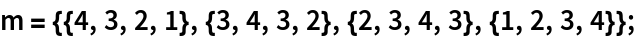Perform the RationalCholeskyDecomposition:

 In:=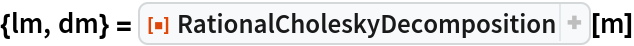Out=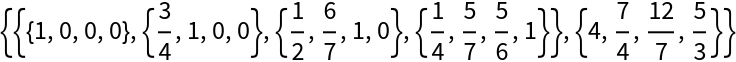Compute the Cholesky decomposition from the result of RationalCholeskyDecomposition:

 In:=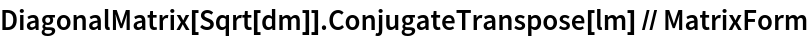Out=Compare with the result of CholeskyDecomposition:

 In:=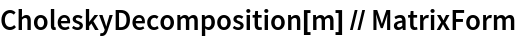Out=## Version History

• 1.0.0 – 06 January 2021